English
English
Home / Biboxpedia / Grid trading logic in depth
Grid trading logic in depth
Service
2020-11-20 09:34

Grid Trading is a type of quantitative trading, and used in traditional finance for many years. It refers to a price based on a certain price, a certain number of prices up and down the price of a certain number of orders, high to sell low to buy.

Operationally, investors divide the price fluctuation range of digital assets into several price levels based on the fluctuation of digital asset prices. The program allocates capital into every price level and pending the maker of buy order.

In practice, when the price of digital assets falls, the buy order is deal and another sell order will be pending at a higher price. Which means that the low buy order corresponds to the high sell order.

When the price of digital assets rises, the sell order is deal and another buy order will be pending at a lower price level. Which means that the low buy order is sold by high price, and then there will be the replenishment soon.

Based on BTC/USDT Trading Pair of Bibox Exchange

You can invest the USDT you hold, then buy BTC at a low price and sell it at a high price（USDT）.

If you want to have a try about the Grid Trading when the BTC price is 10000 USDT, which means that the base price of BTC is P0=10000 USDT.

If you want to buy BTC when there is every 10% reduction of the price, you need to enter 10 into ‘Grid’.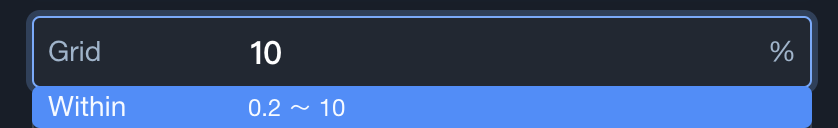The every price level of the buy order is P(n)=P(n-1) ×(1-10%)

That is, the program will

Pending the buy order of BTC in P1, P1=P0×(1-10%)=9000 USDT

Pending the buy order of BTC in P2, P2=P1×(1-10%)=8100 USDT

Pending the buy order of BTC in P3, P3=P2×(1-10%)=7290 USDT

……

If your capital is 10000 USDT, then to be bought in low price, sold in high price.

You need to enter 10000 into ‘USDT Buy Amount’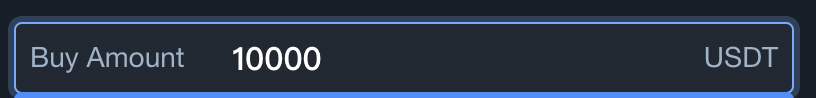If you set the ratio of USDT capital used of each buy order is 20% of the total amount.

You need to enter 20 into ‘Portion’.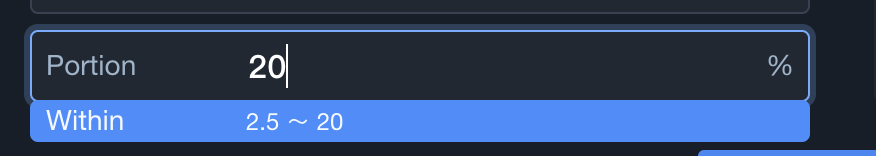The Single buy order amount is USDT Buy Amount * Portion =10000×20%= 2000 USDT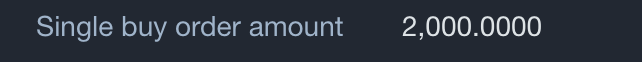The BTC purchase quantity of each order is Single buy order amount /P(n)

That is, the program will

Pending the buy order of BTC as 2000 USDT in P1, approximately equal to single buy order amount/P1=2000/9000≈0.22 BTC

Pending the buy order of BTC as 2000 USDT in P2, approximately equal to single buy order amount/P2=2000/8100≈0.25 BTC

Pending the buy order of BTC as 2000 USDT in P3, approximately equal to single buy order amount/P3=2000/7290≈0.27 BTC

……

When there is the deal of the buy order, the program will pend another sell order at a higher price.

The each price of sell order is P(n)'=P(n)×(1+10%)

That is

When the pending buy order of P1 is deal, then will be another BTC sell order at P1’price, the P1’ is P1×(1+10%)=9900 USDT

When the pending buy order of P2 is deal, then will be another BTC sell order at P2’price, the P2’ is P2×(1+10%)=8910 USDT

When the pending buy order of P3 is deal, then will be another BTC sell order at P3’price, the P3’ is P3×(1+10%)=8019 USDT

……

 USDT Assets operation Buying Rate P(n)（USDT） Selling RateP(n)'（USDT） Quantity Vol(n)（BTC） Profits of each order N(n)（USDT） P0 10,000.00 P1 9,000.00 P1' 9,900.00 Vol1 0.22 N1 200 P2 8,100.00 P2' 8,910.00 Vol2 0.25 N2 200 P3 7,290.00 P3' 8,019.00 Vol3 0.27 N3 200 P4 6,561.00 P4' 7,217.10 Vol4 0.3 N4 200 P5 5,904.90 P5' 6,495.39 Vol5 0.34 N5 200 P(n)=P(n-1)×(1-10%) P(n)'=P(n)×(1+10%) Vol(n)=Single buy order amount/P(n) N(n)=(P(n)'-P(n))×Vol(n) Grid:10%Single buy order amount is USDT Buy Amount * Portion = 2000 USDT

You can invest all the BTC assets you hold, then sell at a high price and buy at a low price.

If you want to have a try about Grid Trading when the price of BTC is 10000 USDT, that is the base price of BTC is P0=10000 USDT.

If you want to sell BTC when there is every 10% increase of the price, you need to enter 10 into ‘Grid’.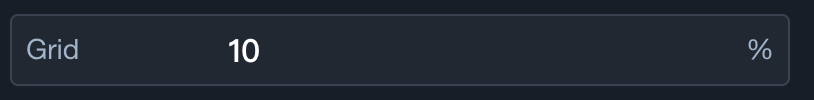The price of sell order is P(n)=P(n-1)×(1+10%)

That is, the program will

Pending the sell order of BTC in P1, P1=P0×(1+10%)=11000 USDT

Pending the sell order of BTC in P2, P2=P1×(1+10%)=12100 USDT

Pending the sell order of BTC in P3, P3=P2×(1+10%)=13310 USDT

……

If your capital is 1 BTC, then to be bought in low price, sold in high price.

You need to enter 1 into ‘BTC Sell Amount’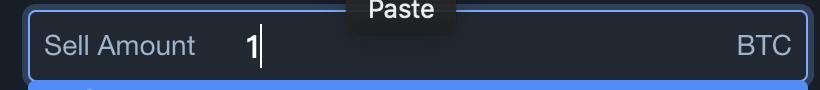If you set the ratio of the BTC capital used of each sell order is 20% of the total amount, you need to enter 20 into ‘Portion’.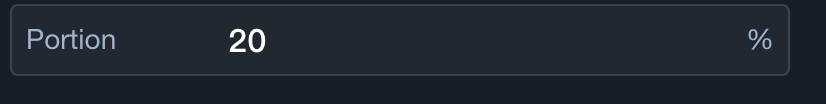The Single sell order quantity is BTC Sell Amount * Portion =1×20%=0.2 BTC

That is, the program will

Pending the sell order of 0.2 BTC in P1

Pending the sell order of 0.2 BTC in P2

Pending the sell order of 0.2 BTC in P3

……

When there is the deal of the sell order, the program will pend another buy order at a lower price.

The each price of buy order is P(n)'=P(n)×(1-10%)

That is

When the sell order of P1 is deal, there will be the buy order of BTC at P1’ price, P1’=P1×(1-10%)=9900 USDT

When the sell order of P2 is deal, there will be the buy order of BTC at P2’ price, P2’=P2×(1-10%)=10890 USDT

When the sell order of P3 is deal, there will be the buy order of BTC at P3’ price, P3’=P3×(1-10%)=11979 USDT

……

 BTC assets operation Selling Rate P (n)（USDT） Cover Price P(n)'（USDT） Quantity Vol(n)（BTC） Profits of each order N(n)（USDT） P5 16,105.10 P5' 14,494.59 Vol5 0.2 N5 322.1 P4 14,641.00 P4' 13,176.90 Vol4 0.2 N4 292.82 P3 13,310.00 P3' 11,979.00 Vol3 0.2 N3 266.2 P2 12,100.00 P2' 10,890.00 Vol2 0.2 N2 242 P1 11,000.00 P1' 9,900.00 Vol1 0.2 N1 220 P0 10,000.00 P(n)=P(n-1)×(1+10%) P(n)'=P(n)×(1-10%) Amount of each sell order N(n)=(P(n)-P(n)')×Vol(n) Grid:10%The Single sell order quantity is BTC Sell Amount * Portion = 0.2 BTC

Grid Trading is a trading method that does not predict the rise or fall. If you strictly follow the Grid Trading method, you will never lose when you sell the digital assets.

As a kind of quantitative trading, the general advantages of Grid trading are as below:

1. The process is completely rational and there will be no panic trading.

2. The process will pend the order automatically.

3. The process will be 24-hour trading and won’t miss the market.

Grid Trading also has the unique advantages:

1. There is no need for users to predict ups and downs, lower the investment threshold.

2. Profits are stable under turbulent market.

--------

You can also contact Bibox telegram admin (@bibox_cs) or join the Telegram group （https://t.me/bibox_trading_bot） for more details.

#### Articles in this section

Return to Bibox website